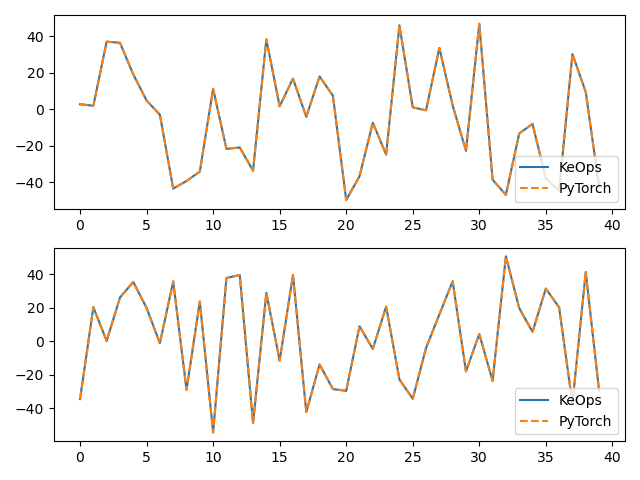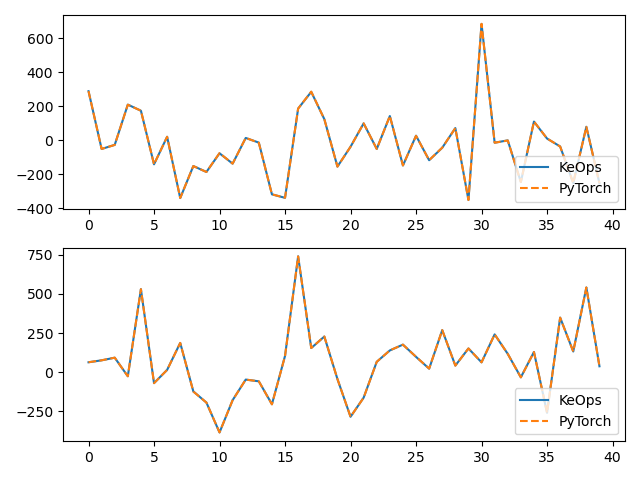# KernelSolve reduction (with LazyTensors)

Let’s see how to solve discrete deconvolution problems using the conjugate gradient solver provided by the `pykeops.torch.LazyTensor.solve()` method of KeOps `pykeops.torch.LazyTensor`.

## Setup

Standard imports:

```import time

import torch
from matplotlib import pyplot as plt

from pykeops.torch import LazyTensor as keops
from pykeops.torch import Vi, Vj
```

Define our dataset:

```N = 5000 if torch.cuda.is_available() else 500  # Number of points
D = 2  # Dimension of the ambient space
Dv = 2  # Dimension of the vectors (= number of linear problems to solve)
sigma = 0.1  # Radius of our RBF kernel

b = torch.rand(N, Dv)
g = torch.Tensor([0.5 / sigma**2])  # Parameter of the Gaussian RBF kernel
alpha = 0.01  # ridge regularization
```

Note

This operator uses a conjugate gradient solver and assumes that formula defines a symmetric, positive and definite linear reduction with respect to the alias `"b"` specified trough the third argument.

Apply our solver on arbitrary point clouds:

```print("Solving a Gaussian linear system, with {} points in dimension {}.".format(N, D))
start = time.time()
K_xx = keops.exp(-keops.sum((Vi(x) - Vj(x)) ** 2, dim=2) / (2 * sigma**2))
cfun = keops.solve(K_xx, Vi(b), alpha=alpha, call=False)
c = cfun()
end = time.time()
print("Timing (KeOps implementation):", round(end - start, 5), "s")
```

Out:

```Solving a Gaussian linear system, with 5000 points in dimension 2.
Timing (KeOps implementation): 0.26499 s
```

Compare with a straightforward PyTorch implementation:

```start = time.time()
K_xx = alpha * torch.eye(N) + torch.exp(
-torch.sum((x[:, None, :] - x[None, :, :]) ** 2, dim=2) / (2 * sigma**2)
)
c_py = torch.solve(b, K_xx)
end = time.time()
print("Timing (PyTorch implementation):", round(end - start, 5), "s")
print("Relative error = ", (torch.norm(c - c_py) / torch.norm(c_py)).item())

# Plot the results next to each other:
for i in range(Dv):
plt.subplot(Dv, 1, i + 1)
plt.plot(c.cpu().detach().numpy()[:40, i], "-", label="KeOps")
plt.plot(c_py.cpu().detach().numpy()[:40, i], "--", label="PyTorch")
plt.legend(loc="lower right")
plt.tight_layout()
plt.show()
```Out:

```/home/code/keops/pykeops/pykeops/examples/pytorch/plot_test_invkernel_torch_helper.py:65: UserWarning:

torch.solve is deprecated in favor of torch.linalg.solveand will be removed in a future PyTorch release.
torch.linalg.solve has its arguments reversed and does not return the LU factorization.
To get the LU factorization see torch.lu, which can be used with torch.lu_solve or torch.lu_unpack.
X = torch.solve(B, A).solution
should be replaced with
X = torch.linalg.solve(A, B) (Triggered internally at  /opt/conda/conda-bld/pytorch_1646755903507/work/aten/src/ATen/native/BatchLinearAlgebra.cpp:859.)

Timing (PyTorch implementation): 0.50277 s
Relative error =  0.00029990272014401853
```

Compare the derivatives:

```print(cfun.callfun)

print("1st order derivative")
e = torch.randn(N, D)
start = time.time()
end = time.time()
print("Timing (KeOps derivative):", round(end - start, 5), "s")
start = time.time()
end = time.time()
print("Timing (PyTorch derivative):", round(end - start, 5), "s")
print("Relative error = ", (torch.norm(u - u_py) / torch.norm(u_py)).item())

# Plot the results next to each other:
for i in range(Dv):
plt.subplot(Dv, 1, i + 1)
plt.plot(u.cpu().detach().numpy()[:40, i], "-", label="KeOps")
plt.plot(u_py.cpu().detach().numpy()[:40, i], "--", label="PyTorch")
plt.legend(loc="lower right")
plt.tight_layout()
plt.show()
```Out:

```<pykeops.torch.operations.KernelSolve object at 0x14d17fdd9310>
1st order derivative
Timing (KeOps derivative): 0.29024 s
Timing (PyTorch derivative): 0.41832 s
Relative error =  0.002301541157066822
```

Total running time of the script: ( 0 minutes 1.737 seconds)

Gallery generated by Sphinx-Gallery